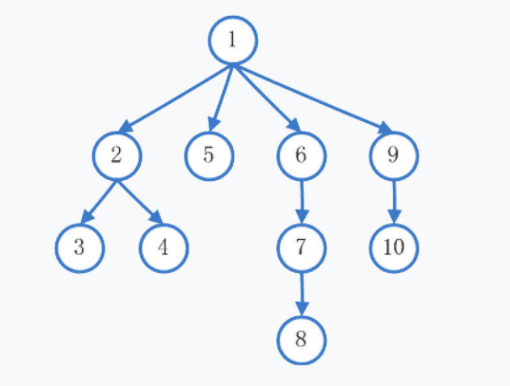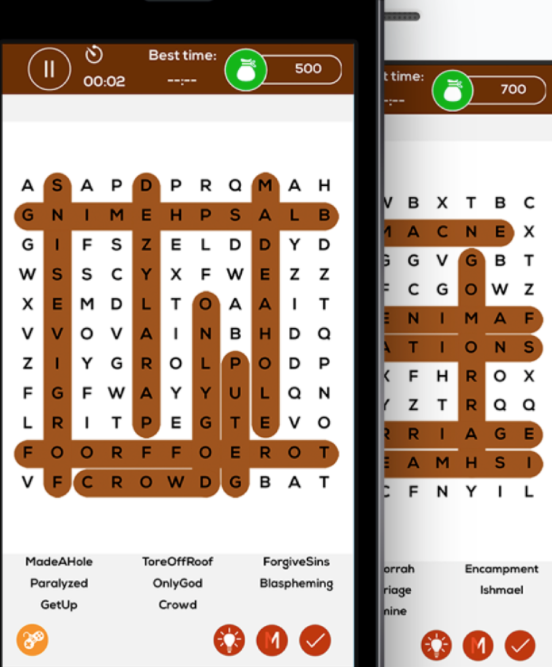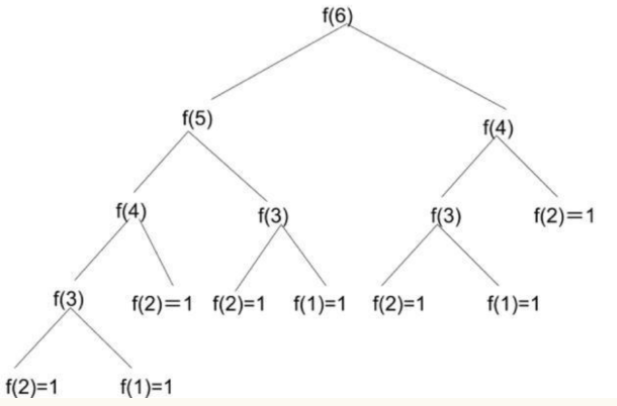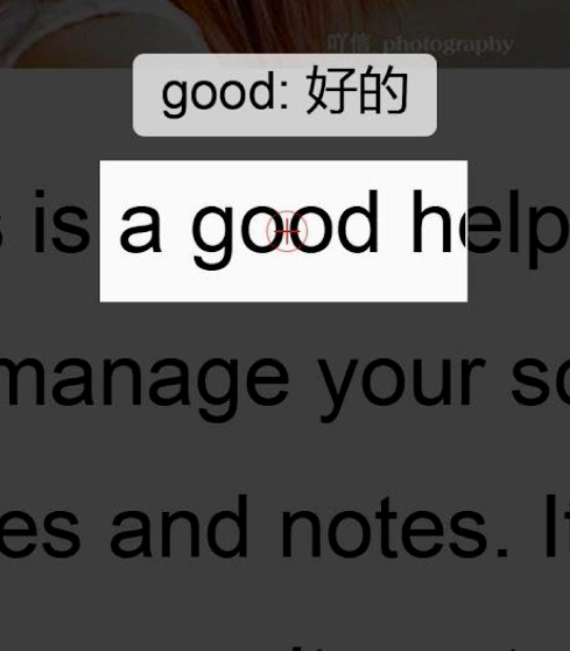# iOS 面试策略之算法基础 6-7 节iOSer# 6. 深度优先和广度优先

• 深度优先搜索（Depth-First-Search，以下简称 DFS）

• 广度优先搜索（Breadth-First-Search，以下简称 BFS）

## 基本概念

DFS 和 BFS 的具体定义这里不做赘述。笔者谈谈自己对此的形象理解：假如你在家中发现钥匙不见了，为了找到钥匙，你有两种选择：

1. 从当前角落开始，顺着一个方向不停的找。假如这个方向全部搜索完毕依然没有找到钥匙，就回到起始角落，从另一个方向寻找，直到找到钥匙或所有方向都搜索完毕为止。这种方法就是 DFS。

2. 从当前角落开始，每次把最近所有方向的角落全部搜索一遍，直到找到钥匙或所有方向都搜索完毕为止。这种方法就是 BFS。DFS 的搜索步骤为：

• 1

• 2 -> 3 -> 4

• 5

• 6 ->7 -> 8

• 9 -> 10

BFS 的搜索步骤为：

• 1

• 2 -> 5 -> 6 -> 9

• 3 -> 4

• 7

• 10

• 8

DFS 的 Swift 实现：

func dfs(_ root: Node?) {    guard let root = root else {        return    }    visit(root)    root.visited = true    for node in root.neighbors {        if !node.visited {            dfs(node)        }    }}

BFS 的 Swift 实现：

func bfs(_ root: Node?) {    var queue = [Node]()    if let root = root {        queue.append(root)    }    while !queue.isEmpty {        let current = queue.removeFirst()        visit(current)        current.visited = true        for node in current.neighbors {            if !node.visited {                queue.append(node)            }        }    }}

## iOS 实战演练var xOffset = 0var yOffset = 0let cellWidth = UIScreen.mainScreen().bounds.size.width / matrix.countlet cellHeight = UIScreen.mainScreen().bounds.size.height / matrix.countfor i in 0 ..< matrix.count {  for j in 0 ..< matrix.count {    let label = UILabel(frame: CGRect(x: xOffset, y: yOffset, width: cellWidth, height: cellHeight))    label.text = String(matrix[i][j])    view.addSubView(label)    labels[i][j] = label    xOffset += cellWidth  }  xOffset = 0  yOffset += cellHeight}

func searchWord(_ board: [[Character]]) -> Bool {    guard board.count > 0 && board.count > 0 else {        return false    }    let (m, n) = (board.count, board.count)    var visited = Array(repeating: Array(repeating: false, count: n), count: m)    for i in 0 ..< m {        for j in 0 ..< n {            if board[i][j] == "c" && dfs(board, Array("crowd"), m, n, i, j, &visited, 0) {                return true            }        }    }    return false}func dfs(_ board: [[Character]], _ wordContent: [Character], _ m: Int, _ n: Int, _ i: Int, _ j: Int, _ visited: inout [[Bool]], _ index: Int) -> Bool {    if index == wordContent.count {        return true    }    guard i >= 0 && i < m && j >= 0 && j < n else {        return false    }    guard !visited[i][j] && board[i][j] == wordContent[index] else {        return false    }    visited[i][j] = true    if dfs(board, wordContent, m, n, i + 1, j, &visited, index + 1) || dfs(board, wordContent, m, n, i - 1, j, &visited, index + 1) || dfs(board, wordContent, m, n, i, j + 1, &visited, index + 1) || dfs(board, wordContent, m, n, i, j - 1, &visited, index + 1) {        return true    }    visited[i][j] = false    return false}

func findWords(_ board: [[Character]], _ dict: Set<String>) -> [String] {}

func findWords(_ board: [[Character]], _ dict: Set<String>) -> [String] {  var res = [String]()  let (m, n) = (board.count, board.count)  let trie = _convertSetToTrie(dict)  var visited = Array(repeating: Array(repeating: false, count: n), count: m)  for i in 0 ..< m {    for j in 0 ..< n {      _dfs(board, m, n, i, j, &visited, &res, trie, "")    }  }  return res}private func _dfs(_ board: [[Character]], _ m: Int, _ n: Int, _ i: Int, _ j: Int, inout _ visited: [[Bool]], inout _ res: [String], _ trie: Trie, _ str: String) {  // 越界  guard i >= 0 && i < m && j >= 0 && j < n else {    return  }  // 已经访问过了  guard !visited[i][j] else {    return  }  // 搜索目前字母组合是否是单词前缀  var str = str + "\(board[i][j])"  guard trie.prefixWith(str) else {    return  }  // 确认当前字母组合是否为单词  if trie.isWord(str) && !res.contains(str) {    res.append(str)  }  // 继续搜索上下左右四个方向  visited[i][j] = true  _dfs(board, m, n, i + 1, j, &visited, &res, trie, str)  _dfs(board, m, n, i - 1, j, &visited, &res, trie, str)  _dfs(board, m, n, i, j + 1, &visited, &res, trie, str)  _dfs(board, m, n, i, j - 1, &visited, &res, trie, str)  visited[i][j] = false}

# 7. 动态规划## 实例讲解

// 此方法会因为栈溢出而崩溃func Fib() -> Int {  var (prev, curr) = (0, 1)  for _ in 1 ..< 100 {    (curr, prev) = (curr + prev, curr)  }  return curr}

1）初始状态，即此问题的最简单子问题的解。在斐波拉契数列里，最简单的问题是，一开始给定的第一个数和第二个数是几？自然我们可以得出是 1；2）状态转移方程，即第 n 个问题的解和之前的 n - m 个问题解的关系。在这道题目里，我们已经有了状态转移方程 F(n) = F(n - 1) + F(n - 2)。

func Fib(_ n: Int) -> Int {  // 定义初始状态  guard n > 0 else {    return 0  }  if n == 1 || n == 2 {    return 1  }  // 调用状态转移方程  return Fib(n - 1) + Fib(n - 2)}print(Fib(100))var nums = Array(repeating: 0, count: 100)func Fib(_ n: Int) -> Int {  // 定义初始状态  guard n > 0 else {    return 0  }  if n == 1 || n == 2 {    return 1  }  // 如果已经计算过，直接调用，无需重复计算  if nums[n - 1] != 0 {    return nums[n - 1]  }  // 将计算后的值存入数组  nums[n - 1] = Fib(n - 1) + Fib(n - 2)  return nums[n - 1]}

• **栈溢出：**每一次递归，程序都会将当前的计算压入栈中。随着递归深度的加深，栈的高度也越来越高，直到超过计算机分配给当前进程的内存容量，程序就会崩溃。

• **数据溢出：**因为动态规划是一种由简至繁的过程，其中积蓄的数据很有可能超过系统 当前数据类型的最大值，从而导致程序抛出异常。

• 首先，递归的次数很多，我们要从 F(100) = F(99) + F(98) ，一直推理到 F(3) = F(2) + F(1)，这样很容易造成栈溢出。

• 其次，F(100) 应该是一个很大的数。实际上 F(40) 就已经突破一亿，F(100) 一定会造成整型数据溢出。

## 斐波拉契数列问题面试实战题**1） 缩小误差范围：**将所有的单词构造成前缀树。然后对于扫描的内容，搜索出相应可能的单词。具体做法可以参考上节《深度优先和广度优先》一文中搜索单词的方法。**2） 计算出最接近的单词：**假如上一步，我们已经有了 10 个可能的单词，那么怎么确定最接近真实情况的单词呢？这里我们要定义两个单词的距离 -- 从第一个单词 wordA，到第二个单词 wordB，有三种操作：

• 删除一个字符

• 添加一个字符

• 替换一个字符

func wordDistance(_ wordA: String, wordB: String) -> Int { ... }

• 删除一个字符：假如已知 wordDistance("abc", "abdf") ，那么 “abce” 只需要删除一个字符到达 “abc” ，然后就可以得知 “abce” 到 “abdf” 之间的距离。

• 添加一个字符：假如已知 wordDistance("abce", "abd")，那么我们只要让 “abd” 添加一个字符到达 “abdf” 即可求出最终解。

• 替换一个字符：假如已知 wordDistance("abc", "abd")，那么就可以依此推出wordDistance("abce", "abde") = wordDistance("abc", "abd")。故而只要将末尾的 “e” 替换成 "f"，就可以得出 wordDistance("abce", "abdf")

• **初始状态：**dp[j] = j，dp[i] = i

• **状态转移方程：**dp[i][j] = min(dp[i - 1][j - 1], dp[i - 1][j], dp[i][j - 1]) + 1

func wordDistance(_ wordA: String, _ wordB: String) -> Int {  let aChars = Array(wordA), bChars = Array(wordB)  let aLen = aChars.count, bLen = bChars.count  var dp = Array(repeating: (Array(repeating: 0, count: bLen + 1)), count: aLen + 1)  for i in 0 ... aLen {    for j in 0 ... bLen {      // 初始情况      if i == 0 {        dp[i][j] = j      } else if j == 0 {        dp[i][j] = i      // 特殊情况      } else if aChars[i - 1] == bChars[j - 1] {        dp[i][j] = dp[i - 1][j - 1]      } else {        // 状态转移方程        dp[i][j] = min(dp[i - 1][j - 1], dp[i - 1][j], dp[i][j - 1]) + 1      }    }  }  return dp[aLen][bLen]}

# 推荐👇：## 评论